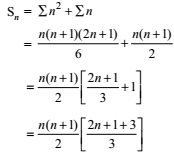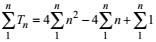>

## NCERT Solutions for Class 11 Maths Chapter 9 Sequence and Series Exercise 9.4

If you're in search of NCERT Solutions of Chapter 9 Sequence and Series Exercise 9.4 then you can find them here. We at Studyrankers have prepared detailed and accurate Class 11 Maths NCERT solutions of every questions so you can easily get them at one place without wasting your precious time. These will help you a lot if you want to improve your marks in the examinations.1. Find the sum of the following series upto n terms:
1 × 2 + 2 × 3 + 3 × 4 + 4 × 5 + .....

Let Tn denotes the nth term of the given series
Tn = [nth term of 1, 2, 3, ......] × [nth term of 2, 3, 4, .....]
= [1 + (n – 1) 1] [2 + (n – 1)1]
= n (n + 1)
Tn = n 2 + n= (n(n +1)(2n + 4))/6
= (2n(n + 1)(n + 2))/6 = (n(n + 1)(n + 2))/3

2. Find the sum of the following series upto n terms: 1 × 2 × 3 + 2 × 3 × 4 + 3 × 4 × 5 + .......

Let Tn denote the nth term of the given series.
Then
Tn = [nth term of 1, 2, 3, ......] [n th term of 2, 3, 4, .....] [n th term of 3, 4, 5, .....]
= [1 + (n – 1)1] [2 + (n – 1).1] [3 + (n –1).1]
= n (n + 1) (n + 2)
= n(n 2 + 2n + 3) = n 3 + 3n 2 + 2n
∴ Sn = ∑n3 + 3∑n2 + 2∑n
= (n2(n + 1)2)/4 + (3n(n + 1)(2n +1))/6 + 2.(n(n+1))/2
= (n(n + 1))/4 [n(n + 1) + 2(2n + 1) + 4]
= (n(n + 1))/4 [n2 + n + 4n + 2 + 4]
= (n(n + 1))/4 (n2 + 5n + 6)
= (n(n + 1)(n + 2)(n + 3))/4

3. Find the sum of n terms of the following series.
3 × 12 + 5 × 22 + 7 × 32 + .....

Let Tn denote the nth term of the given series.
Then,
Tn = [nth term of 3, 5, 7,...] [nth term of 1, 2, 3, ...]2
= {3 + 2 (n – 1)2}{1 + (n – 1).1]2
= (2n + 1) (n)2
= n 2 (2n + 1) = 2n 3 + n 2
Sn = 2∑n+ ∑n2
= 2. (n2(n + 1)2)/4 + (n(n + 1)(2n + 1))/6
= (n(n + 1))/6 . [3n(n + 1) + (2n + 1)]
= (n(n + 1))/6 (3n2 + 3n + 2n + 1)
= (n(n+1)(3n2 + 5n + 1))/6

4. Find the sum of n terms of the following series. 1/(1 × 2) + 1/(2 × 3) + 1/(3 × 4) + …………

Tn = 1/([nth term of 1, 2, 3, …..][n th term of 2, 3, 4, ….])
= 1/([1 + (n -1)1][2 + (n – 1)1]) = 1/(n(n + 1))
Let 1/(n(n + 1)) = A/n + B/(n + 1) ……….(1)
=> 1 = A(n + 1) + Bn …………(2)
[on multiplying both sides by n(n + 1)]
To find A: Put n = 0 in (2), we get
1 = A(0 + 1) => A = 1
To find B: put n = -1 in (2), we get
1 = B(-1) => B = -1
Put these values of A and B in (1), the partial fractions are
1/(n(n + 1)) = 1/n – 1/(n + 1)
∴ Tn = 1/n – 1/(n + 1)
Putting n = 1, 2, 3, ………n, we get,
T1 = 1/1 – 1/2
T2 = 1/2 – 1/3
T3 = 1/3 – 1/4
……………………..
Tn = 1/n – 1/(n + 1)
Sn = 1 – 1/(n + 1) = (n + 1 – 1)/(n + 1) = n/(n + 1)

5. Find the sum of the following series upto n terms:
5 2+ 62 + 72 +.... 202

Tn of given series
= (nth term of 5, 6, 7, ....)2
= [5 + (n + 1) × 1]2
= (n + 4)2 = n 2 + 8n + 16
Sn = ∑n2 + 8∑n + 16 × n
= (n(n + 1)(2n + 1))/6 + 8 × (n(n + 1))/2 + 16n
= n/6[(n + 1)(2n + 1) + 24(n + 1) + 96]
= n/6[2n2 + n + 2n + 1+ 24n + 24 + 96]
= n/6 (2n2 + 27n + 121)
Put n = 16, then
S = 16/6 ( 2 × 16+ 27 × 16 + 121)
8/3 (512 + 432 + 121) = 8/3 × 1065
= 8 × 355 = 2840
Let Tn = 20, a = 5
d = 1
=> 20 = 5 + (n –1).1
or 20 = 5 + n – 1
or 20 – 4 = n
or n = 16

6. Find the sum of the following series upto n terms
3 × 8 + 6 × 11 + 9 × 14 +....

Here the series is formed by multiplying the corresponding terms of two series both of which are A.P.
viz. 3, 6, 9, ...... and 8, 11, 14, ......
Tn of given series = (nth term of 3, 6, 9,...) × (nth term of 8, 11, 14,...)
= [3 + (n –1)3] [8 + (n – 1)3]
= [3n] [3n + 5] = 9n 2 + 15n
Sn = 9∑n2 + 15 ∑n
= 9 × (n(n + 1)(2n + 1))/6 + (15.n(n + 1))/2
= 3/2 . n(n + 1)[2n + 1 + 5]
= 3/2 . n(n + 1)(2n + 6) = 3n(n + 1)(n + 3)

7. Find the sum to n terms of given series, 1 2 + (12 + 22) + (12 + 22 + 32) + ................

Let Tn denote the n th term, then
Tn = 12 + 22 + 32 + ..........+ n 2
= ∑n2 = (n(n + 1)(2n + 1))/6
= 1/6 (2n3 +3n2 + n)
S= 1/6 [2 ∑n3 + 3 ∑n2 + ∑n]
= 1/6 [ 2. (n2 (n + 1)2)/4 + 3. (n(n +1 )(2n + 1))/6 + (n(n + 1))/2]
= (n(n + 1))/12 [n(n + 1) + (2n + 1) + 1]
= (n(n + 1))/12 [n2 + n + 2n + 1+ 1]
= (n(n + 1)(n2 + 3n + 2))/12 = (n(n + 1)(n + 1)(n + 2))/12
= (n(n + 1)2(n + 2))/12

8. Find the sum to n terms of the following series whose nth term is given by:
n(n + 1) (n + 4)

Tn = n(n + 1) (n + 2) = n(n 2 + 5n + 4)
= n 3 + 5n 2 + 4n
Sn == [(n(n + 1)2)/2] + 5. (n(n + 1)(2n + 1))/6 + 4 . (n(n + 1))/2
= (n2(n + 1)2)/4 + (5n(n + 1)(2n + 1))/6 + 2n(n + 1)
= (n(n + 1))/12 [3n(n + 1) + 10(2n + 1) + 2n]
= (n(n + 1))/12 [3n2 + 3n + 20n + 10 + 2n]
= (n(n + 1))/12 [3n2 + 23n + 3n]
= 1/12 . n(n + 1)[3n+ 23n + 3n]

9. Find the sum to n terms of the following series whose n th term is given by:
n 2 + 2n

Tn = n 2 + 2n
Put n = 1, 2, 3, ....., n, we get,
T1 = 12 + 21
T2 = 22 + 22
T3 = 32 + 23
.........................
Tn = n 2 + 2n
Sn = (12 + 22 + 32 +... + n 2) + (21 + 22 + 23 +... + 2n)
= ∑n2 + (2(2n – 1))/(2 - 1)
= (n(n + 1)(2n + 1))/6 + 2(2n – 1)

10. Find the sum to n terms of the given series whose n th term is (2n –1)2.

Sn =